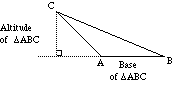index: click on a letter A B C D E F G H I J K L M N O P Q R S T U V W X Y Z A to Z index index: subject areas numbers & symbols sets, logic, proofs geometry algebra trigonometry advanced algebra & pre-calculus calculus advanced topics probability & statistics real world applications multimedia entrieswww.mathwords.com about mathwords website feedback

 Altitude of a Triangle Height of a Triangle The distance between a vertex of a triangle and the opposite side. Formally, the shortest line segment between a vertex of a triangle and the (possibly extended) opposite side. Altitude also refers to the length of this segment. Note: The three altitudes of a triangle are concurrent, intersecting at the orthocenter.See also